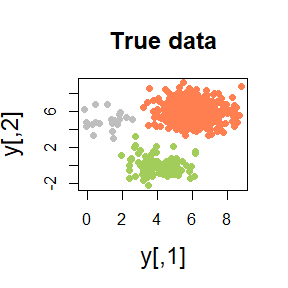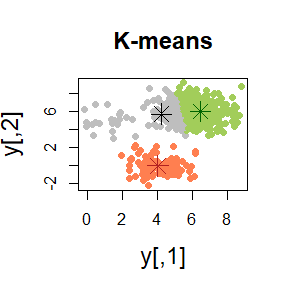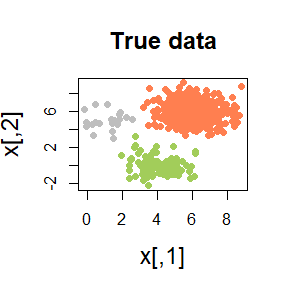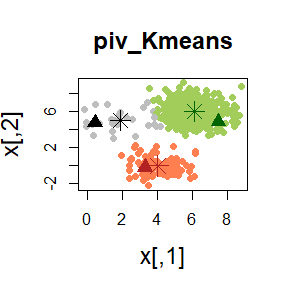# K-means clustering using MUS and other pivotal algorithms

#### 2019-02-26

In this vignette we explore the K-means algorithm performed using the MUS algorithm and other pivotal methods through the function piv_KMeans of the pivmet package. First of all, we load the package:

library(pivmet)

## Pivotal algorithms: how they works, and why

We present here a simulated case for applying our procedure. Given $$n$$ units $$y_1,\ldots,y_n$$:

• consider to build a co-association matrix $$C$$, by taking the co-occurrences of pairs of $$n$$ units in the same cluster/group among the total number of partitions. For instance, this matrix could be constructed from a MCMC output arising from Bayesian mixture models;

• suppose we want to detect the pivotal units, as the observations that are as far away from each other as possible according to the co-association matrix. Units which are very distant from each other are likely to have zero co-occurrences;

• the resulting units—hereafter pivots—have the desirable property to be representative of the group they belong to.

We propose four alternative methods for achieving this task. Let $$j$$ be the group containing units $$\mathcal J_j$$, the user may choose $${i^*}\in\mathcal J_j$$ that maximizes one of the quantities:

\begin{align*} & \sum_{p\in\mathcal J_j} c_{{i^*}p} \\ & \sum_{p\in\mathcal J_j} c_{{i^*}p} - \sum_{j\not\in\mathcal J_j} c_{{i^*}p}. \end{align*}

These methods give the unit that maximizes the global within similarity (maxsumint) and the unit that maximizes the difference between global within and between similarities (maxsumdiff), respectively. Alternatively, we may choose $$i^{*} \in\mathcal J_j$$, which minimizes:

$\sum_{p\not\in\mathcal J_j} c_{i^{*}p},$

obtaining the most distant unit among the members that minimize the global dissimilarity between one group and all the others (minsumnoint).

MUS algorithm described in Egidi et al. (2018a) is a sequential procedure for extracting identity submatrices of small rank and pivotal units from large and sparse matrices. The procedure has already been satisfactorily applied for solving the label switching problem in Bayesian mixture models (Egidi et al. 2018b).

With the function MUS the user may detect pivotal units from a co-association matrix C, obtained through $$H$$ different partitions, whose units may belong to $$k$$ groups, expressed by the argument clusters. We remark here that MUS algorithm may be performed only when $$k <5$$.

#generate some data

set.seed(123)
n  <- 620
centers  <- 3
n1 <- 20
n2 <- 100
n3 <- 500
x  <- matrix(NA, n,2)
truegroup <- c( rep(1,n1), rep(2, n2), rep(3, n3))

for (i in 1:n1){
x[i,]=rmvnorm(1, c(1,5), sigma=diag(2))}
for (i in 1:n2){
x[n1+i,]=rmvnorm(1, c(4,0), sigma=diag(2))}
for (i in 1:n3){
x[n1+n2+i,]=rmvnorm(1, c(6,6), sigma=diag(2))}

H <- 1000
a <- matrix(NA, H, n)

for (h in 1:H){
a[h,] <- kmeans(x,centers)$cluster } #build the similarity matrix sim_matr <- matrix(1, n,n) for (i in 1:(n-1)){ for (j in (i+1):n){ sim_matr[i,j] <- sum(a[,i]==a[,j])/H sim_matr[j,i] <- sim_matr[i,j] } } cl <- KMeans(x, centers)$cluster
mus_alg <- MUS(C = sim_matr, clusters = cl, prec_par = 5)

## piv_KMeans

Quite often, classical K-means fails in recognizing the true groups:In such situations, we may need a more robust version of the classical K-means. The pivots may be used as initial seeds for a classical K-means algorithm. The function piv_KMeans works as the classical kmeans function, with some optional arguments

piv_res <- piv_KMeans(x, centers)The function piv_KMeans has optional arguments:

• piv.criterion: one among the four different pivotal criteria described above and listed in Egidi et al. (2018b): MUS, maxsumint, minsumnoint, maxsumdiff. MUS is the default criterion when centers $$\le$$ 4, whereas maxsumdiff is the default method when centers > 4.

• iter.mus: the number of different partitions used for building the co-association matrix $$C$$. Default is $$10^3$$.

• prec.par: with this argument the user may increase the powerful of the underlying MUS algorithm (see Egidi et al. (2018a) for details). The usual choice is $$\min\{ \underset{k}{\min} \ n_{k}-1, 5 \}$$, where $$n_k$$ is the number of units belonging to the group $$k, \ k=1,\ldots,K$$.

• alg.type: the clustering technique applied to the raw data such that the partition is given in input to the MUS algorithm. A robust choice is required here—by default, KMeans is used.